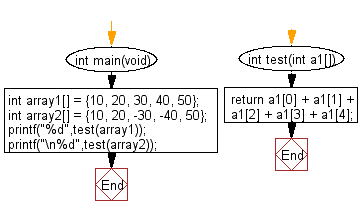﻿ C Program: Sum the elements of an array of integers# C Exercises: Compute the sum of the elements of a given array of integers

## C-programming basic algorithm: Exercise-36 with Solution

Write a C program to compute the sum of the elements of a given array of integers.

C Code:

``````#include <stdio.h>
#include <stdlib.h>
int main(void){
int array1[] = {10, 20, 30, 40, 50};
int array2[] = {10, 20, -30, -40, 50};
printf("%d",test(array1));
printf("\n%d",test(array2));
}
int test(int a1[])
{
return a1 + a1 + a1 + a1 + a1;
}
``````

Sample Output:

```150
10
```

Pictorial Presentation:Flowchart:C Programming Code Editor:

What is the difficulty level of this exercise?

Test your Programming skills with w3resource's quiz.

﻿

## C Programming: Tips of the Day

Why doesn't a+++++b work?

printf("%d",a+++++b); is interpreted as (a++)++ + b according to the Maximal Munch Rule!.

++ (postfix) doesn't evaluate to an lvalue but it requires its operand to be an lvalue.

! 6.4/4 says the next preprocessing token is the longest sequence of characters that could constitute a preprocessing token"

Ref : https://bit.ly/3fdldUT